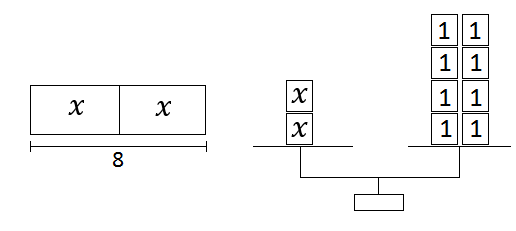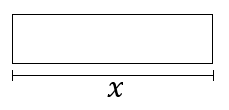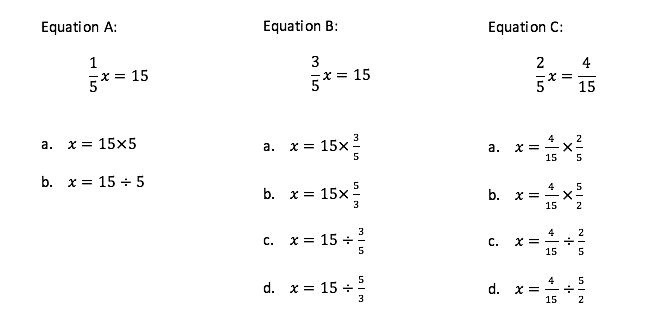Match Fishtank is now Fishtank Learning!

# Equations and Inequalities

## Objective

Solve one-step equations with multiplication and division.

## Common Core Standards

### Core Standards

?

• 6.EE.B.6 — Use variables to represent numbers and write expressions when solving a real-world or mathematical problem; understand that a variable can represent an unknown number, or, depending on the purpose at hand, any number in a specified set.

• 6.EE.B.7 — Solve real-world and mathematical problems by writing and solving equations of the form x + p = q and px = q for cases in which p, q and x are all nonnegative rational numbers.

?

• 5.NF.B.3

• 5.NF.B.4

• 6.NS.A.1

• 6.NS.B.2

## Criteria for Success

?

1. Understand that in the process of solving for a variable, whatever is done to one side of the equation must also be done to the other side in order to maintain the balance.
2. Use models and diagrams to solve for a variable.
3. Solve one-step multiplication and division equations algebraically.
4. Write and solve one-step multiplication and division problems for real-world contexts.
5. Interpret ${a/b}$ as $a$ divided by $b$, and where $b$ is a fraction, as $a$ multiplied by the reciprocal of $b$

## Tips for Teachers

?

In this lesson, students encounter problems that draw on skills from the Number Sense domain, namely dividing by fractions and decimals. Students may need to review or recall these concepts in order to fully access this lesson.

#### Remote Learning Guidance

If you need to adapt or shorten this lesson for remote learning, we suggest prioritizing Anchor Problems 1and 2 (benefit from worked examples). Find more guidance on adapting our math curriculum for remote learning here.

#### Fishtank Plus

• Problem Set
• Student Handout Editor
• Vocabulary Package

## Anchor Problems

?

### Problem 1

The following two diagrams represent the equation ${ 2x=8}$. Explain how you can use each one to find the value of $x$.How can you solve the equation ${3.4m=13.6}$ without using a diagram?

### Problem 2

Complete the tape diagram below to represent the equation ${{x\over5}=10}$; then use it to find the value of x.How can you solve the equation ${{p\over6}=9}$ without using a diagram?

### Problem 3

Three equations are shown below. For each one, identify all of the ways that equation could be solved.## Problem Set

?The following resources include problems and activities aligned to the objective of the lesson that can be used to create your own problem set.

• Include problems with procedural practice in solving multiplication and division problems.
• Include problems where students write an equation from a context (any operation, but focused on multiplication and division) and solve the equation.
• Include error analysis problems of mistakes made in solving equations.
• Include equations where students must divide a fraction by a fraction.

?

### Problem 1

Ron solved the equation ${ {{{2\over3}}}r=12}$ by multiplying both sides by ${{2\over3}}$ and he got ${r=8}$. Explain the error that Ron made and then find the correct value for $r$.

### Problem 2

Lee filled several jars with ${{1\over4}}$ cup of water in each jar. He used a total of 8 cups of water. Let $j$ represent the number of jars that Lee filled.

Write and solve an equation to find out how many jars Lee filled.

?